# Lines of Symmetry on Plane Shapes | Vertical and Horizontal Lines | Cardinal Points | Types of Angles Primary 4 (Basic 4) Term 3 Week 9 Mathematics

### MATHEMATICS

THIRD TERM

WEEK 9

PRIMARY 4

THEME – MENSURATION AND GEOMETRY

PREVIOUS LESSON – Capacity – Multiplication and Division in Litres and Millilitres Primary 4 (Basic 4) Term 3 Week 8 Mathematics

### TOPIC – LINES AND ANGLES

#### LEARNING AREA

1. Introduction

2. Lines of Symmetry

3. Vertical and Horizontal Lines

4. Meaning and Types of Angles

5. Cardinal Points

6. Lesson Evaluation and Weekly Assessment (Test)

#### LEARNING OBJECTIVES

By the end of the lesson, most pupils should have attained the following objectives –

1. describe the lines of symmetry on plane shapes.

2. identify the symmetrical lines on plane shapes such as triangles, squares and rectangles.

3. locate lines of symmetry of plane figures at school and home.

4. differentiate between vertical and horizontal lines.

6. identify the four cardinal points.

7. identify the right angles, acute and obtuse angles of plane shapes.

#### ENTRY BEHAVIOUR

The pupils can differentiate between different types of lines.

#### INSTRUCTIONAL MATERIALS

The teacher will teach the lesson with the aid of pencil, ruler, compass, protractor, plain paper, chart showing cardinal points, different types of lines and angles, etc.

#### METHOD OF TEACHING

Choose a suitable and appropriate methods for the lessons.

Note – Irrespective of choosing methods of teaching, always introduce an activities that will arouse pupil’s interest or lead them to the lessons.

#### REFERENCE MATERIALS

1. Scheme of Work

2. 9 – Years Basic Education Curriculum

3. Course Book

4. All Relevant Material

5. Online Information

### CONTENT OF THE LESSON

#### LESSON 1 – INTRODUCTION

ACTIVITY 1 – MEANING OF LINES

A line is a straight or curved mark made by marking materials like pen, pencil, etc.

A line can be thin or thick line. When two or more lines are combined, a shape, image or sign is formed.

Line is one of the important elements of design.

ACTIVITY 2 – TYPES OF LINES

1. Vertical lines

2. Horizontal lines

3. Diagonal lines

4. Zigzag lines

5. Curved lines

6. Dotted lines

7. Dash lines

8. Parallel line

9. Perpendicular line

1. VERTICAL LINES

Vertical lines are straight lines that move up and down without any slant.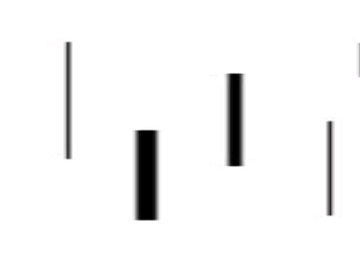2. HORIZONTAL LINES

Horizontal lines are straight lines that are parallel to the horizon.3. DIAGONAL LINES

Diagonal lines are straight thin or thick lines that slant.4. ZIGZAG LINES

Zigzag lines are straight thin or thick lines made from a combination of diagonal lines.5. CURVED LINES

Curved lines are thin or thick lines that change direction gradually.6. DOTTED LINES

Dotted lines are lines made up of a series of dots.7. DASH LINES

Dotted lines are lines made up of a series of dots.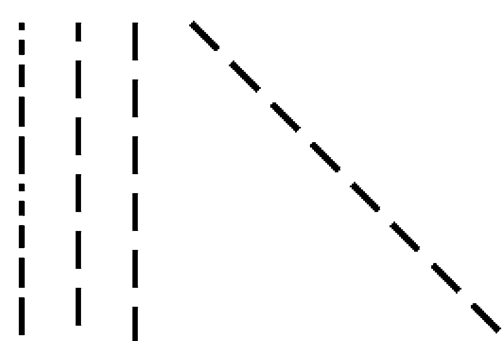8. PARALLEL LINES

Parallel lines are lines in a plane that are always the same distance apart like equal sign.

### ______________________

9. PERPENDICULAR LINES

Perpendicular lines are lines that intersect at right angles.ACTIVITY 3 – USES/IMPORTANCE OF LINES

The uses of lines are as follows:

1. It is used to indicate a part.

2. It is used show direction.

3. It is used as a symbol.

4. It is used as a plan.

5. It is used to make a sketch.

6. It is used to mark out.

7. It is used to cut out a part.

8. It is used as measurement.

ACTIVITY 4 – DIFFERENT BETWEEN VERTICAL AND HORIZONTAL LINES

A vertical line is the straight line that goes top to bottom or bottom to top without any slant while horizontal line is the straight lines that goes from left to right or right to left without any slant.

CLASS EXERCISE

1. Explain the meaning of lines.

2. state and draw types of lines.

3. differentiate between parallel and perpendicular lines.

4. what I’d the different between vertical and horizontal lines.

5. state 5 uses of lines.

#### LESSON 2 – LINES OF SYMMETRY

ACTIVITY 1 – INTRODUCTION

A line of symmetry is a line that a shape exactly into two.

For example,

If you fold a shape such a square into two and both parts would match exactly, the line in between is known as line of symmetry.

ACTIVITY 2 – FOLDING SHAPES WITH 3 SIDES OR MORE

Teacher organizes pupils in groups or pairs depending on the size of the class to determine the lines of symmetry by folding or cutting.

1. Triangles

There are 3 major types of triangles,

• Equilateral triangle has 3 lines of symmetry.
• Isosceles triangle has one line of symmetry.
• Scalene Triangle has zero line of symmetry.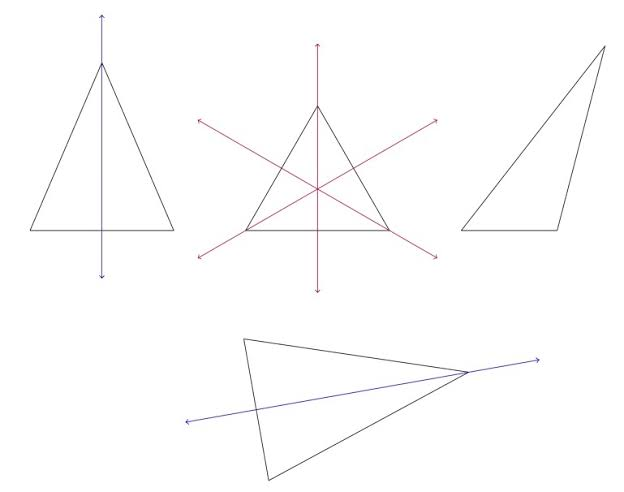There are different types of quadrilateral are as follows:

• Square has 4 lines of symmetry.
• Rectangle has 2 lines of symmetry.
• Rhombus has 2 lines of symmetry.
• Parallelogram has no line of symmetry.
• Trapezium has no line of symmetry.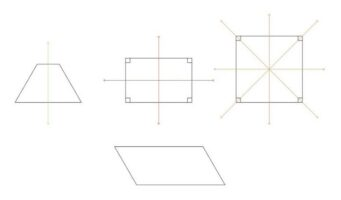#### LESSON 3 – MEANING AND TYPES OF ANGLES

ACTIVITY 1 – GROUP WORK

Materials Needed – Plain sheet of papers, rulers and pencil.

Teacher organizes pupils in groups or pairs depending on the size of the class to carry out the following instructions,

• Draw a straight line.
• Draw another straight line from the starting or end point of the first line.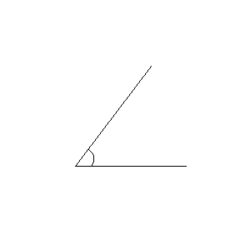CONCEPT OF ANGLE

An angle is the space between two intersecting lines.

ACTIVITY 2 – GROUP WORK

Materials Needed – Plain sheet of papers, protractors, rulers, pencil chart showing different types of angles such as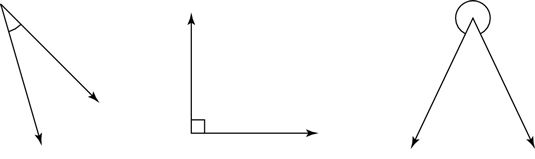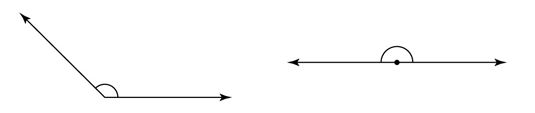Teacher uses the existing groups or pairs and guides them to measure and record correctly each of the above angle.

Record,

1.

2.

3.

4.

5.

ACTIVITY 3 – TYPES OF ANGLES

An angle is the space between two intersecting lines.

There are different types of angles,

1. Right angle

2. Acute angle

3. Obtuse angle

4. Straight angle or angle on a straight line

5. Reflex angle

6. Angle on a point

7. Complimentary angle

8. Supplementary angle

1. RIGHT ANGLE

Right angle is the angle that forms a square corner.

It is an angle that is always equal 90°.

2. ACUTE ANGLE

Acute angle is any angle that less than 90°.

It is an angle between 1° – 90°.

Acute angle is greater 0° and less than 90°.

3. OBTUSE ANGLE

Obtuse angle is any angle that is larger (bigger) than right angle.

It is an angle between 91° – 179°.

Obtuse is greater than greater 90° and less than 180°.

4. STRAIGHT ANGLE

Straight angle is any angle that is equal to 180°.

It is twice of right angles.

Straight angle is equal to 180°.

5. REFLEX ANGLE

Reflex angle is any angle that is greater than 180° and less than 360°.

6. ANGLE AT A POINT

Angel at a point is any angle that is equal to 360°.

7. COMPLIMENTARY ANGLES

Complimentary angles are two angles that are sum up to 90º.

Two angles are said be complimentary angles, when they add up to 90°.

8. SUPPLEMENTARY ANGLE

Supplementary angles are two angles that are sum up to 180º.

Two angles are said be supplementary angles, when they add up to 180°.

#### LESSON 4 – CARDINAL POINTS (BEARING)

ACTIVITY 1 – OUTDOOR ACTIVITIES

Teacher’s activities,

• regroups the pupils or maintains the existing groups or pairs.
• marks the primary and secondary cardinal points on the field.
• lets 8 of the pupils represent the primary and secondary cardinal points.
• guides the groups or pair to locate some of the things or objects such as car, trees, flowers, stones, drainage, other buildings inside and outside school, animals, etc. starting from north position.

Pupil’s Activities – Identify living and non living within and around the school with their locations or directions.

ACTIVITY 2 – CARDINAL POINTS

Bearing is the direction along which someone or something moves or faces.

It is used to describe or determine the position of someone or something.

Sometimes, it is used as right , left, forward (up) or backward (down).

Geographically, North, South, East and West are used in describing bearing.

These positions are known as cardinal points.

North, East, West and South are called primary points or positions while North East (NE), North West (NW), South East (SE) and South West (SW).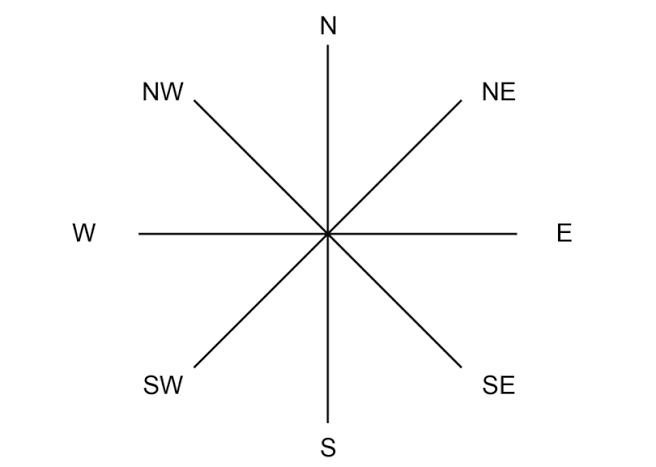ACTIVITY 3 – CLASSROOM ACTIVITIES

Teacher marks the four all walls of the classroom as the North, East, South and West and lets pupils find the location of,

• all the doors, if there are more than one door.
• all bulbs, if there are more than one bulb.
• all the windows, if there are more than one wind.
• all the pupils are front, back, right, left and in between.

LESSON 5 – REVISION AND WEEKLY ASSESSMENT (TEST)

#### PRESENTATION

To deliver the lesson, the teacher adopts the following steps:

1. To introduce the lesson, the teacher revises the previous lesson. Based on this, he/she asks the pupils some questions;

2. Teacher organizes the pupils in groups or pair depending on the size of the class.

3. Teacher displays chart showing different types of lines.

4. Teacher asks pupils to compare and different between the lines.

Pupil’s Activities – Compare and identify different between the lines.

5. Teacher listens to the pupils and uses their responses to the introduce the lesson.

Pupil’s Activities – Pay attention to the lesson introduction to understand the concept of the lesson.

6. Teacher leads a discussion on the meaning, types and uses of lines.

Pupil’s Activities – Identify and differentiate between different types of lines.

7. Teacher displays both triangles, squares and rectangles.

8. Teacher guides the group or pairs to fold and cut each shape into two.

Pupil’s Activities – Carefully fold or cut each shape into two and compare.

9. Teacher uses pupil’s activity in steps 8 to introduce and discuss the lines of symmetry.

Pupil’s Activities – Identify and describe each property of a circle.

Teacher guides pupils draw lines to form angels.

Pupil’s Activities – Use lines for form angles.

10. Teacher uses the pupils drawings to discuss the meaning and types of angles with appropriate examples from pupil’s drawings.

11. Teacher guides pupils to differentiate between the types of angles using appropriate examples.

Pupil’s Activities – Identify and describe each type of angle.

12. Teacher leads a outdoor activities to introduce and discuss the concepts of bearing and to locate living and non things around.

13. Teacher continues the bearing activities in the classroom to find the location of different things in the class.

Pupil’s Activities – Participate actively in the outdoor and indoor activities on bearing.

14. Teacher guides pupils to find the location of some angles using the cardinal points and to draw and indicate angles on the cardinal points.

Pupil’s Activities – Find the location of some angles using the cardinal points and to draw and indicate angles on the cardinal points.

15. Teacher leads a working exercises on measuring angles, supplementary and complimentary angles.

Pupil’s Activities – Participate actively in the working exercises.

16. Teacher summarizes each of the lesson on the board with appropriate evaluation.

Pupil’s Activities – Participate actively in the summary of the lesson by responding correctly in the questions and write as instructed.

### CONCLUSION

To conclude the lesson for the week, the teacher revises the entire lesson and links it to the following week’s lesson.

### NEXT LESSON

Three Dimensional (3D)Shapes | Meaning and Properties of Three Dimensional (3D) Shapes | Different between 2D and 3D Shapes Primary 4 (Basic 4) Term 3 Week 10 Mathematics

#### LESSON EVALUATION

1. describe the lines of symmetry on plane shapes.

2. identify the symmetrical lines on plane shapes such as triangles, squares and rectangles.

3. state the number of symmetrical lines in,

• equilateral triangle
• isosceles triangle
• scalene triangle
• square
• rectangle

4. indicate the symmetrical lines of the following shapes,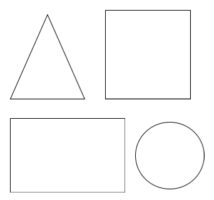5. locate lines of symmetry of plane figures at school and home such as the football field, surface of classroom floor, walls, book, door, etc.

4. differentiate between vertical and horizontal lines with appropriate drawing.

6. state the four cardinal points.

7. use diagram to describe the following angles,

• right angle
• acute angle
• obtuse angles

8. with the aid of diagram, differentiate between acute and obtuse angels with appropriate examples.

THANKS FOR USING CLASSROOMNOTE!!!